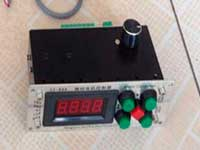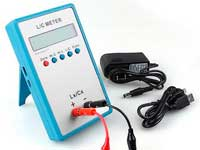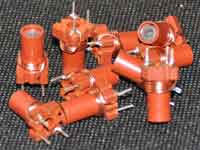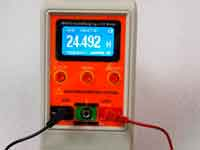Pi-matching network calculator - 5.0 out of 5 based on 1 vote
Rating 5.00 (1 Vote)

Online calculators

Pi-matching network calculator:

The calculator is designed to calculate a Pi-matching circuit of a transmitter power amplifier. It is assumed that the idle Q-factor of C1 and C2 is much higher than that of the L. It is true for most capacitors. Increasing the loaded Q-factor of the Pi-circuit Qp, increases harmonic suppression and condensers capacitance (important for high-power electron tubes), but reduces efficiency.

For the output Pi-network of the amplifier:
R1 ≈ Ea / (2.1 • Ia)
(where Ea is a constant voltage and Ia is a direct current of the anode), or
R1 ≈ (0.9Ea) 2 / 2P
(where P is the input power of the matching circuit).

In case of input Pi-matching circuit for the power amplifier with a common grid
R2 ≈ 1 / S
(where S is the gain slope of the electron tube).

To estimate the design requirements for the circuit parts, effective currents in the coil and in the capacitors are calculated. For capacitors, peak voltage and reactive power are also calculated. The peek voltage can increase 2..3 times (depending on the antenna SWR) compared with the exact matching case.

Pi-matching network calculator
Put your own values F, QL, QP  (for tubes  10...20,  for transistors 2...10), Рin, R1, R2.
 Working frequency F [MHz] Q-factor of the coil; QL Q-factor of the Pi-network; QP Input power Рin [W]

 L: [µH]
 Source impedance R1 [Ohm]Load impedance R2 [Ohm]
 C1: [pF] C2: [pF]

 Reactive Power Pr [VA] Peek Voltage Umax [V]] Peek Current Imax [A] С1 С2

 Peek current in the L [А]: Output power Рout [W]: Losses in the L [W]: Efficiency factor %:

Original calculator by DL2KQ is the prototype of this calculator.

The calculator has been developed by DL2KQ collaborating with UA3DA UA3SDE and other.

Сomments from anonymous guests are enabled with moderation.

Coil Winding MachineUS \$187.53
The system consists of individual components including a controller, a stepping motor, a foot switch, power adapter and output shaft. This design makes it very easy for you to integrate the system into your own project.

LC Meter LC200AUS \$37.99
Measuring Capacitance Range:
0.01 pF - 100mF
Measuring Inductance Range:
0.001 uH - 100H
Measurement accuracy:1%.

RF InductorsUS \$6.43
Variable Ferrite Core Inductor coil set 3.5Turns 25-100MHZ

Auto Ranging LCR MeterUS \$63.07
Meter can measure 0.00pF to 100.00mF capacitance, 0.000uH to 100.00H inductance and 0.00? to 10.000M? resistance. Very suitable for small value capacitor and inductor measurement.